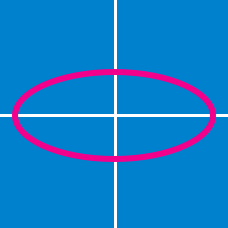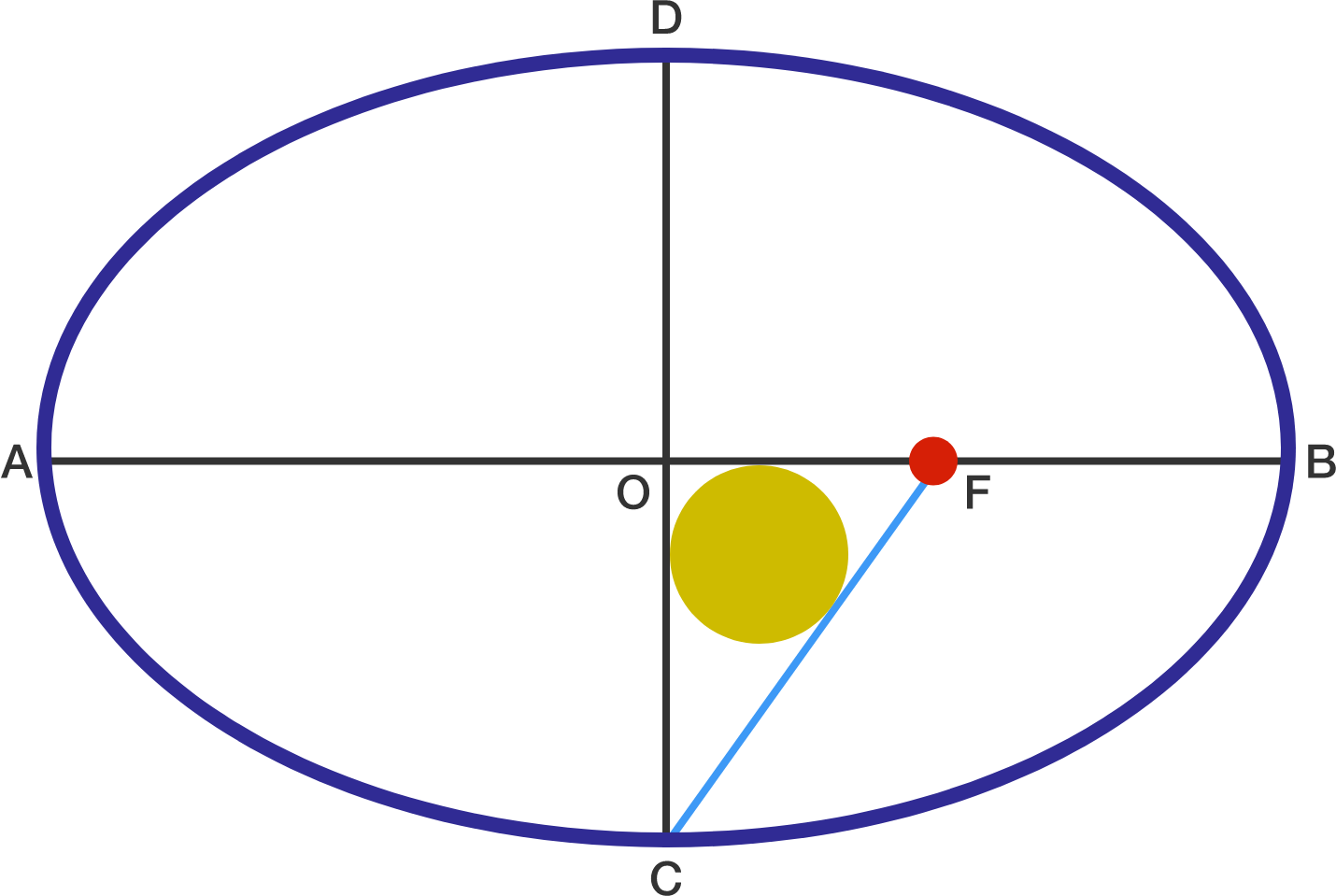Geometry

# Conic Sections: Level 2 Challenges

What is the number of intersections between $y=4$ and $x^2+y^2=9$?

For any real number $\alpha$, the parabola $f_\alpha (x) = 2x^2 + \alpha x + 3 \alpha$ passes through the common point $(a, b)$. What is the value of $a+b?$

Consider the point $P(-1, 0)$ on the ellipse given by the equation $4x^2 + y^2 = 4$. There are two points $(a, b)$ and $(a, c)$ on the ellipse whose distance from $P$ is a maximum. What is the value of $a$?Point $O$ is the center of the ellipse with major axis $AB$ & minor axis $CD$ Point F is one of the focus of this ellipse.

If $OF=6$, and the diameter of inscribed circle of triangle $\triangle{OCF}$ is $2$, then find $(AB)\cdot (CD)$

There are four lines that are tangent to both circles
${ x }^{ 2 }+{ y }^{ 2 }=1 \quad \text{ and } \quad ({ x }-6)^{ 2 }+{ y }^{ 2 }=4.$

What is the sum of the slopes of these four lines?

×